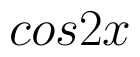Select Page

# CBSE MCQ Maths 12 Science Inverse Trigonometric Functions Answers in English

CBSE MCQ Maths 12 Science Inverse Trigonometric Functions Answers in English to enable students to get Answers in a narrative video format for the specific question.

Expert Teacher provides CBSE MCQ Maths 12 Science Inverse Trigonometric Functions Answers through Video Answers in English language. This video solution will be useful for students to understand how to write an answer in exam in order to score more marks. This teacher uses a narrative style for a question from Inverse Trigonometric Functions not only to explain the proper method of answering question, but deriving right answer too.

Please find the question below and view the Answer in a narrative video format.

Question:

Answer Video in English:

You can select video Answers from other languages also. Please check Answers in ( Hindi )

## Similar Questions from CBSE, 12th Science, Maths, Inverse Trigonometric Functions

Question 1 :Then x is equal to : (View Answer Video)

Question 2 : Write the principal value of(View Answer Video)

Question 3 :is equal to: (View Answer Video)

Question 4 : Solve for(View Answer Video)

Question 5 : Write the value of(View Answer Video)

### Application of Derivatives

Question 1 : The slope of the tangent to the curveat the point (2,-1) is _______________. (View Answer Video)

Question 2 : Find the approximate change in volume V of a cube of side x meters caused by increasing the side by 1%. (View Answer Video)

Question 3 : Find two numbers whose sum is 24 and whose product is as large as possible. (View Answer Video)

Question 4 : A balloon, which always remains spherical has a variable radius. Find the rate at which its volume is increasing with the radius when the later is 10 cm.  (View Answer Video)

Question 5 : A cylindrical tank of radius 10 m is being filled with wheat at the rate of the 314 cubic meters per hour. Then the depth of the wheat is increasing at the rate of ___________. (View Answer Video)

### Integrals

Question 1 : Find :(View Answer Video)

Question 2 : Prove that :(View Answer Video)

Question 3 : Write an antiderivative for the function,using the method of inspection. (View Answer Video)

Question 4 : Find the integral of the function. (View Answer Video)

Question 5 : Evaluate :. (View Answer Video)

### Three Dimensional Geometry

Question 1 : Find the distance between the planes 2x+3y+4z=10 and 4x+6y+8z=18. (View Answer Video)

Question 2 : Write the equation of the straight line through the pointand parallel to z-axis. (View Answer Video)

Question 3 : If the equation of a line AB isthen, find the direction cosines of a line parallel to AB. (View Answer Video)

Question 4 : Find the distance between the point (5, 4, -6) and its image in xy-plane. (View Answer Video)

Question 5 : Find the equation of the plane with intercept 3 on the y-axis and parallel to ZOX plane. (View Answer Video)